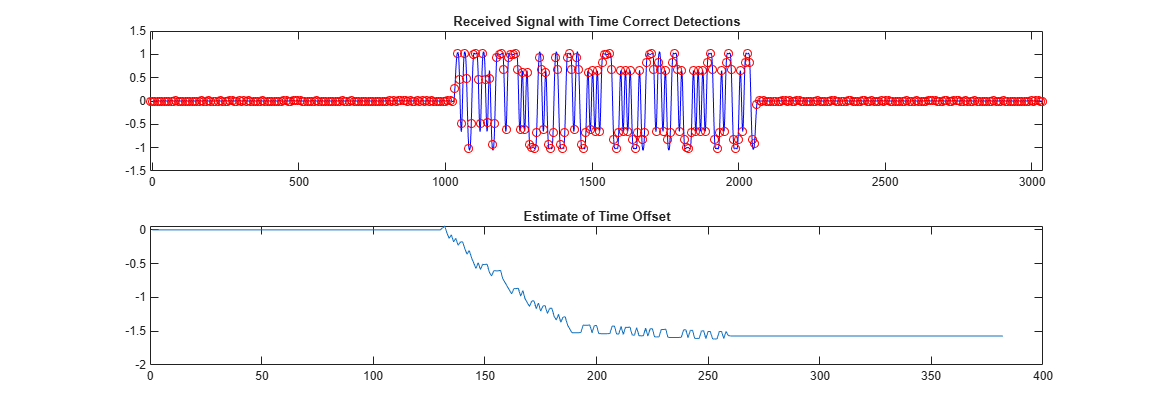# Timing Offset Estimation

This example shows how to generate HDL code from a basic lead-lag timing offset estimation algorithm implemented in MATLAB® code.

### Introduction

In wireless communication systems, receive data is oversampled at the RF front end. This serves several purposes, including providing sufficient sampling rates for receive filtering.

However, one of the most important functions is to provide multiple sampling points on the received waveform such that data can be sampled near the maximum amplitude point in the received waveform. This example illustrates a basic lead-lag time offset estimation core, operating recursively.

The generated hardware core for this design operates at 1/os_rate where os_rate is the oversampled rate. That is, for 8 oversampled clock cycles this core iterates once. The output is at the symbol rate.

```design_name = 'mlhdlc_comms_toe'; testbench_name = 'mlhdlc_comms_toe_tb'; ```

Let us take a look at the MATLAB® design.

```type(design_name); ```
```%%%%%%%%%%%%%%%%%%%%%%%%%%%%%%%%%%%%%%%%%%%%%%%%%%%%%%%%%%%%%%%%%%%%%%%%%%% % MATLAB design: Time Offset Estimation % %% Introduction: % % The generated hardware core for this design operates at 1/os_rate % where os_rate is the oversampled rate. That is, for 8 oversampled clock cycles % this core iterates once. The output is at the symbol rate. % % Key design pattern covered in this example: % (1) Data is sent in a vector format, stored in a register and accessed % multiple times % (2) The core also illustrates basic mathematical operations % % Copyright 2011-2015 The MathWorks, Inc. %#codegen function [tauh,q] = mlhdlc_comms_toe(r,mu) persistent tau persistent rBuf os_rate = 8; if isempty(tau) tau = 0; rBuf = zeros(1,3*os_rate); end rBuf = [rBuf(1+os_rate:end) r]; taur = round(tau); % Determine lead/lag values and compute offset error zl = rBuf(os_rate+taur-1); zo = rBuf(os_rate+taur); ze = rBuf(os_rate+taur+1); offsetError = zo*(ze-zl); % update tau tau = tau + mu*offsetError; tauh = tau; q = zo; ```
```type(testbench_name); ```
```function mlhdlc_comms_toe_tb % % Copyright 2011-2015 The MathWorks, Inc. os_rate = 8; Ns = 128; SNR = 100; mu = .5; % smoothing factor for time offset estimates % create simulated signal rng('default'); % always default to known state b = round(rand(1,Ns)); d = reshape(repmat(b*2-1,os_rate,1),1,Ns*os_rate); x = [zeros(1,Ns*os_rate) d zeros(1,Ns*os_rate)]; y = awgn(x,SNR); w = fir1(3*os_rate+1,1/os_rate)'; z = filter(w,1,y); r = z(4:end); % give it an offset to make things interesting %tau = 0; Nsym = floor(length(r)/os_rate); tauh = zeros(1,Nsym-1); q = zeros(1,Nsym-1); for i1 = 1:Nsym-1 rVec = r(1+(i1-1)*os_rate:i1*os_rate); % Call to the Timing Offset Estimation Algorithm [tauh(i1),q(i1)] = mlhdlc_comms_toe(rVec,mu); end indexes = 1:os_rate:length(tauh)*os_rate; indexes = indexes+tauh+os_rate-1-os_rate*2; Fig1Loc=figposition([5 50 90 40]); H_f1=figure(1); clf; set(H_f1,'position',Fig1Loc); subplot(2,1,1) plot(r,'b'); hold on plot(indexes,q,'ro'); axis([indexes(1) indexes(end) -1.5 1.5]); title('Received Signal with Time Correct Detections'); subplot(2,1,2) plot(tauh); title('Estimate of Time Offset'); function y=figposition(x) %FIGPOSITION Positions figure window irrespective of the screen resolution % Y=FIGPOSITION(X) generates a vector the size of X. % This specifies the location of the figure window in pixels % screenRes=get(0,'ScreenSize'); % Convert x to pixels y(1,1)=(x(1,1)*screenRes(1,3))/100; y(1,2)=(x(1,2)*screenRes(1,4))/100; y(1,3)=(x(1,3)*screenRes(1,3))/100; y(1,4)=(x(1,4)*screenRes(1,4))/100; ```

### Simulate the Design

It is always a good practice to simulate the design with the testbench prior to code generation to make sure there are no runtime errors.

```mlhdlc_comms_toe_tb ```### Create a New HDL Coder™ Project

```coder -hdlcoder -new mlhdlc_toe ```

Next, add the file `mlhdlc_comms_toe.m` to the project as the MATLAB Function and `mlhdlc_comms_toe_tb.m` as the MATLAB Test Bench.

Refer to Get Started with MATLAB to HDL Workflow for a more complete tutorial on creating and populating MATLAB HDL Coder projects.

### Run Fixed-Point Conversion and HDL Code Generation

Launch the Workflow Advisor from the Workflow Advisor button and right-click on the Code Generation step and choose the option Run to selected task to run all the steps from the beginning through the HDL code generation.

Examine the generated HDL code by clicking on the hyperlinks in the Code Generation Log window.